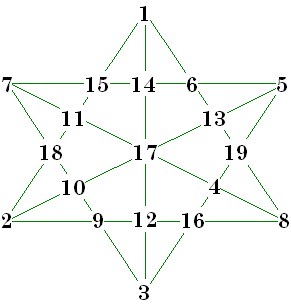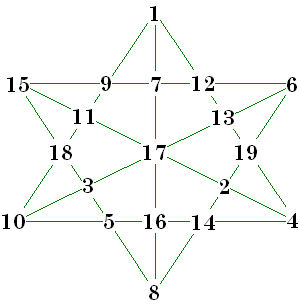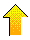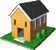# Magic 9x5 HexagonsS = 46  Magic Stars are normally considered to be figures of 4 numbers in a row that add to the magic constant. The magic hexagram is such a pattern, and consists of the numbers 1 to 12 arranged in 6 lines of 4 numbers such that each line sums to the constant S. The sum of the series 1 to 12 is 78, there are 6 lines with each cell appearing in 2 lines, so the magic constant (S) is (2 x 78)/6 = 26. 

In 1989, Harold Reiter and David Richie considered an elaboration of this figure .
Suppose 3 additional lines were added to the hexagram (from point to opposite point). If a number (in the series 1 to 19) is placed at each point of intersection, there will be 5 numbers on each of the 9 lines. Can these numbers be arranged in such a manner that all 9 lines total to the same value?

They found that the answer was affirmative. Their algorithm and the resulting computer search program found all possible solutions. There are actually 9 separate magic constants, ranging from 46 to 54.

This search problem was made manageable by using several short-cuts.

1. Search for solutions with the magic constant from 46 to 50 only. Solutions for S= 51 to 54 are obtained from these by complementing the numbers (i.e. subtract each number from 20). When S=50 the complement is another solution with S=50.
2. Consider only a standard position solution when searching. No need to consider the other 31 rotations or reflections (which are considered equivalent in magic objects).

Their search involved only 18,264,704 checks instead of the 19! that would have been required if all combinations were checked.

The number of basic solutions are:

 46 91 47 284 48 377 49 888 50 1100 51 888 52 377 53 284 54 91 Total 4380

 http://www.geocities.com/~harveyh/order6.htm
 Harold Reiter and David Richie , A Complete Solution to the Magic Hexagon Problem, College Mathematics Journal, Vol. 20:4, 1989, pages 307-316.S=47S = 48

The S = 51 solution below is the complement of the S = 49 solution shown.S = 49S = 50S = 51Please send me Feedback about my Web site!Harvey Heinz   harveyheinz@shaw.ca